# Adding different numbers using various Creative Questions

In this quiz, you will learn how to add various numbers as well as how to identify the given addition statement from the given questions. Addition is a basic skill that every student must know hence it is important for every student to clear the addition concepts at an early age. Various types of questions are provided in the quiz in a visually creative way to make the quiz more interactive and engaging.

Start Quiz

Find the sum of given numbers and choose the correct option.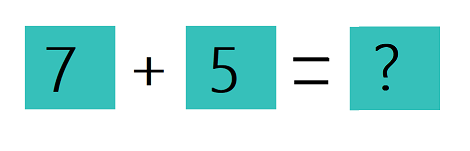11

8

12

15

Fill in the blanks to write the correct addition sentence for the given picture.

(For example 3 + 4 = 7)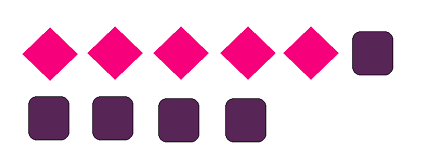##5## + ##5## = 10

5 5

Find the sum of given numbers and choose the correct option.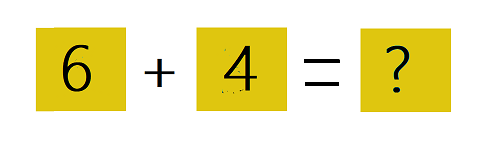12

13

10

8

Find the sum of given numbers and choose the correct option.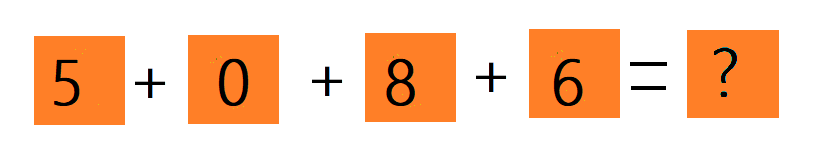17

13

19

15

Fill in the blanks to write the correct addition sentence for the given picture.

(For example 3 + 4 = 7)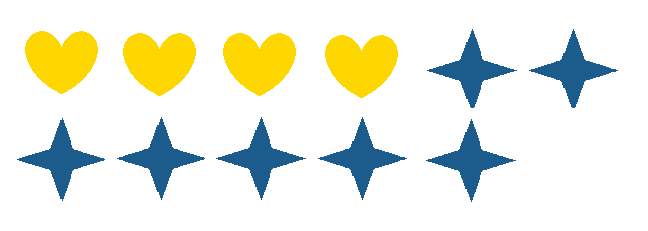##4## + 7 = ##11##

11 4

Find the sum of given numbers and choose the correct option.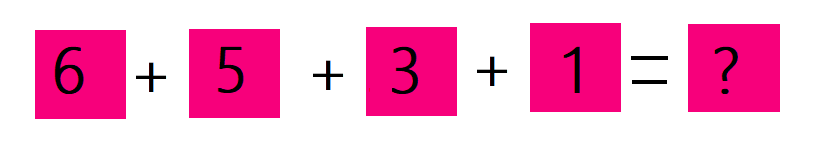18

19

12

15

Choose the correct option to balance the given equation.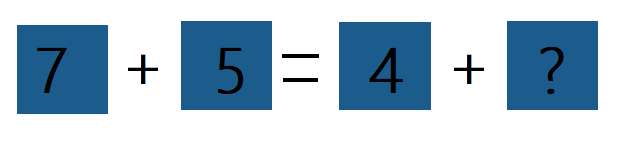3

4

8

12

Find the correct sum of given numbers.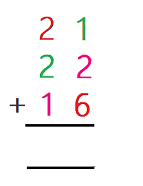34

54

59

45

Fill in the blanks to write the correct addition sentence for the given picture.

(For example 3 + 4 = 7)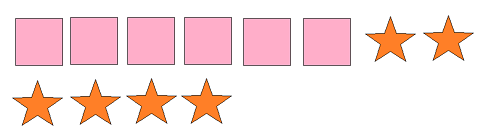6 + ##6## = ##12##

6 12

Choose the correct option to balance the given equation.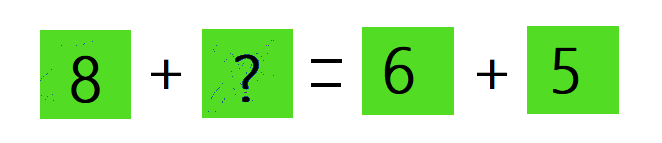3

11

5

13

Quiz/Test Summary
Title: Adding different numbers using various Creative Questions
Questions: 10
Contributed by: